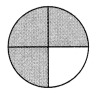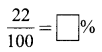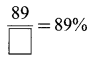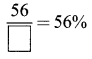# DAV Class 5 Maths Chapter 12 Brain Teasers Solutions

The DAV Books Solutions Class 5 Maths and DAV Class 5 Maths Chapter 12 Brain Teasers Solutions of Percentage offer comprehensive answers to textbook questions.

## DAV Class 5 Maths Ch 12 Brain Teasers Solutions

Question 1.
(a) 10% of 74.6 is-
(i) 4.6
(ii) 7.4
(iii) 7.46
(iv) 746
Solution:
$$\frac{10}{100}$$ of 74.6 = $$\frac{10}{100}$$ × 74.6 = 7.46
So, option (iii) is correct.

(b) 8 tenths = ______%
(i) 8
(ii) 80
(iii) 0.8
(iv) 0.08
Solution:
8 tenth = ($$\frac{8}{10}$$ × 100)% = 80%
So, option (ii) is correct.

(c) 33$$\frac{1}{3}$$% of 10% of 600 litres = ______ litres.
(i) 20
(ii) 120
(iii) 200
(iv) 220
Solution:
33$$\frac{1}{3}$$% of 10% of 600 l
= $$\frac{100}{3}$$% × 10% × 600 l
= $$\frac{100}{3} \times \frac{1}{100} \times \frac{10}{100}$$ × 600 l
= 20 l
So, option (i) is correct.(d) The percentage of shaded part in the given figure is-(i) 80%
(ii) 70%
(iii) 75%
(iv) 85%
Solution:
$$\frac{3}{4}$$ = ($$\frac{3}{4}$$ × 100)% = 75%
So, option (iii) is correct.

(e) Jack went up the hill and came down with a bucket three-fifth filled with water. Then the percentage of water left to be filled in the bucket is-
(i) 60%
(ii) 30%
(iii) 40%
(iv) 50%
Solution:
$$\frac{3}{5}$$ = ($$\frac{3}{5}$$ × 100)%
= (3 × 20)%
= 60%
% of water left to be filled = (100 – 60)% = 40%
So, option (iii) is correct.

Question 2.
Write each of the following as a percentage.
(a) 7 tenths
Solution:
7 tenths = ($$\frac{7}{10}$$ × 100)% = 70%

(b) $$\frac{328}{100}$$
Solution:
$$\frac{328}{100}$$ = ($$\frac{328}{100}$$ × 100)% = 328%

(c) 0.07
Solution:
0.07 = ($$\frac{0.07}{100}$$ × 100)% = 7%

(d) 5$$\frac{1}{5}$$
Solution:
5$$\frac{1}{5}$$ = $$\frac{26}{5}$$
= ($$\frac{26}{5}$$ × 100)%
= 520%Question 3.
Express as a fraction in the lowest term.
(a) 35%
Solution:
35% = $$\frac{35}{100}$$ = $$\frac{7}{20}$$

(b) 16$$\frac{2}{3}$$%
Solution:
16$$\frac{2}{3}$$% = $$\frac{50}{3 \times 100}$$ = $$\frac{1}{6}$$

(c) 2$$\frac{3}{4}$$%
Solution:
2$$\frac{3}{4}$$% = $$\frac{11}{4} \times \frac{1}{100}$$ = $$\frac{11}{400}$$

(d) 90%
Solution:
90% = $$\frac{90}{100}$$ = $$\frac{9}{10}$$

Question 4.
Express as a decimal.
(a) 71%
Solution:
71% = $$\frac{71}{100}$$ = 0.71

(b) 9.5%
Solution:
9.5% = $$\frac{9.5}{100}$$ = 0.095

(c) 101%
Solution:
101% = $$\frac{101}{100}$$ = 1.01

(d) 759%
Solution:
759% = $$\frac{759}{100}$$ = 7.59

Question 5.
Find the value of-
(a) 33$$\frac{1}{3}$$% of 429 metres
Solution:
33$$\frac{1}{3}$$% of 429 metres
= $$\frac{100}{3 \times 100}$$ × 429
= 143 m

(b) 50% of 4 metres
Solution:
50% of 4 metres
= $$\frac{50}{100}$$ × 4
= 2 m(c) 100% of 200 grams
Solution:
100% of 200 grams
= $$\frac{100}{100}$$ × 200
= 200 gm

(d) 20% of 1 kg
Solution:
20% of 1 kg
= $$\frac{20}{100}$$ × 1
= $$\frac{1}{5}$$
= 0.2 kg or 200 gms

(e) 6$$\frac{1}{4}$$% of 1 litre
Solution:
6$$\frac{1}{4}$$% of 1 litre
= $$\frac{25}{4 \times 100}$$ × 1
= $$\frac{1}{16}$$ l
= 0.0625 l

(f) 12$$\frac{1}{2}$$% of ₹ 32
Solution:
12$$\frac{1}{2}$$% of ₹ 32
= $$\frac{25}{2 \times 100}$$ × 32
= ₹ 4

Question 6.
A school contributed ₹ 3,500 to the flood relief fund. Sonal donated 10% of the fund. How much money was donated by Sonal?
Solution:
Total contribution of school = ₹ 3,500
Sonal’s contribution = 10%
Money contribution of Sonal = $$\frac{10}{100}$$ × 3500 = ₹ 350
The money donated by Sonal was ₹ 350.

Question 7.
Mr Arun earns ₹ 35,500 per month. He spends 70% on household expenses, 25% on children, and saves the rest. How much money did Arun save?
Solution:
Earning of Mr Aran per month = ₹ 35,500
His expenditure = 70% + 25% = 95%
Saves = (100 – 95)% = 5%
His savings = $$\frac{5}{100}$$ × 35500 = ₹ 1,775
Arun saved ₹ 1,775.Question 8.
Mr Gupta took his family out for dinner. The amount of the bill was ₹ 875. Mr Gupta paid 5% of the bill amount as a tip. Find the money given as a tip.
Solution:
Amount of bill = ₹ 875
% of tip = 5%
Money as tip = 5% of ₹ 875
Amount paid at tip = $$\frac{5}{100}$$ × 875 = ₹ 43.75
The money given as tip is ₹ 43.75.

Question 9.
Find the percentage of the letter ‘M’ in the word MATHEMATICS.
Solution:
Total alphabets = 11
% of ‘M’ = ($$\frac{2}{11}$$ × 100)%
= ($$\frac{200}{11}$$)%
= 18$$\frac{2}{11}$$%

Question 10.
Some numbers are below:
5, 2, 18, 26, 31, 42, 65, 7, 13, 35
Find the percentage of:
(a) Even numbers
(b) Odd numbers
(c) Prime numbers
(d) Odd primes
(e) Multiples of 5
(f) Even primes
Solution:
(a) Total numbers = 10
Even numbers = 4
% of even numbers = ($$\frac{4}{10}$$ × 100)% = 40%

(b) Total numbers = 10
Odd numbers = 6
% of odd numbers = ($$\frac{6}{10}$$ × 100)% = 60%

(c) Total number = 10
Prime numbers = 5
% of prime numbers = ($$\frac{5}{10}$$ × 100)%

(d) Total numbers = 10
Odd prime numbers = 4
% of odd primes = ($$\frac{4}{10}$$ × 100)% = 40%

(e) Total numbers = 10
Multiples of 5 = 3
% of multiples of 5 = ($$\frac{3}{10}$$ × 100)% = 30%(f) Total numbers = 10
Even primes = 1
% of even primes = ($$\frac{1}{10}$$ × 100)% = 10%

Question 1.
Complete the following:
(a)Solution:
$$\frac{22}{100}$$ = 22%

(b)Solution:
$$\frac{133}{100}$$ = 133%

(c)Solution:
$$\frac{46}{100}$$ = 46%

(d)Solution:
$$\frac{89}{100}$$ = 89%

(e)Solution:
$$\frac{56}{100}$$ = 56%Question 2.
Convert the following percentages into fractions in the lowest term:
(a) 45%
Solution:
45% = $$\frac{45}{100}$$ = $$\frac{9}{20}$$

(b) 125%
Solution:
125% = $$\frac{125}{100}$$
= $$\frac{5}{4}$$
= 1$$\frac{1}{4}$$

(c) 176%
Solution:
176% = $$\frac{176}{100}$$
= $$\frac{44}{25}$$
= 1$$\frac{19}{25}$$

(d) 89%
Solution:
89% = $$\frac{89}{100}$$

Question 3.
Express the following percentages into decimals:
(a) 8.8%
Solution:
8.8% = $$\frac{8.8}{100}$$ = 0.088

(b) 8.9%
Solution:
8.9% = $$\frac{8.9}{100}$$ = 0.089

(c) 2.9%
Solution:
2.9% = $$\frac{2.9}{100}$$ = 0.029

(d) 0.38%
Solution:
0.38% = $$\frac{0.38}{100}$$ = 0.0038

Question 4.
Convert the following into percentages.
(a) $$\frac{8}{25}$$
Solution:
$$\frac{8}{25}$$ = ($$\frac{8}{25}$$ × 100)% = 32%

(b) 3$$\frac{1}{4}$$
Solution:
3$$\frac{1}{4}$$ = ($$\frac{13}{4}$$ × 100)%
= (13 × 25)%
= 325%

Question 5.
Convert the following into percentages.
(a) 0.732
Solution:
0.732 = (0.732 × 100)% = 73.2%

(b) 3.7
Solution:
3.7 = (3.7 × 100)% = 370%

(c) 31.87
Solution:
31.87 = (31.87 × 100)% = 3187%

(d) 41.35
Solution:
41.35 = (41.35 × 100)% = 4135%Question 6.
Find
(a) 20% of 800
Solution:
20% of 800 = $$\frac{20}{100}$$ × 800 = 160

(b) 2$$\frac{1}{4}$$% of 160
Solution:
2$$\frac{1}{4}$$% of 160 = $$\frac{9}{4}$$ × $$\frac{1}{100}$$ × 160 = 3.6

(c) 2% of 400
Solution:
2% of 400 = $$\frac{2}{100}$$ × 400 = 8